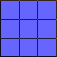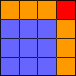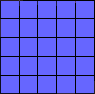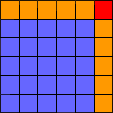How do I go about finding a formula for the number of tiles I would need to add to an arbitrary square to get to the next sized square? Hi Rosa, Get some squares or graph paper and try it. Don't look for a formula but rather watch what you do. Here is a 3 by 3 square.Make it into a 4 by 4 square.Now try a 5 by 5 squareand make it a 6 by 6 square.In each case you add a row on the top (orange), a column on the right (orange) and one more tile (red). That is, for a 3 by 3 square you add 3 + 3 + 1 tiles and for the 5 by 5 square you add 5 + 5 + 1 tiles. For an arbitrary square, say one that is n by n, how many tiles do you add? Penny Go to Math Central